Courses

# NCERT Solutions(Part- 1)- Mensuration Class 8 Notes | EduRev

## Mathematics (Maths) Class 8

Created by: Full Circle

## Class 8 : NCERT Solutions(Part- 1)- Mensuration Class 8 Notes | EduRev

The document NCERT Solutions(Part- 1)- Mensuration Class 8 Notes | EduRev is a part of the Class 8 Course Mathematics (Maths) Class 8.
All you need of Class 8 at this link: Class 8

Question: (a) Match the following figures with their respective areas in the box.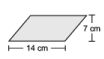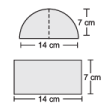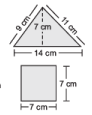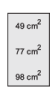(b) Write the perimeter of each shape.

Solution: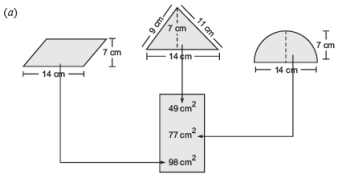(b) (i) The given figure is a rectangle in which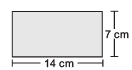Length = 14 cm

∵ Perimeter of a rectangle = 2 * [Length + Breadth]

∴ Perimeter of the given figure = 2 * [14 cm + 7 cm]

= 2 * 21 cm = 42 cm

(ii) The figure is a square housing its side as 7 cm.

∵ Perimeter of a square = 4 * Side

∴ Perimeter of the given figure = 4 * 7 cm = 28 cm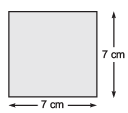Exercise 11.1

Question 1. A square and a rectangular field with measurements as given in the figure have the same perimeter. Which field has a larger area?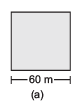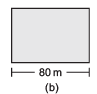Solution:

(a) Side of the square = 60 m

∴ Its perimeter = 4 * Side

= 4 * 60 m = 240 m

Area of the square = Side * Side

= 60 m * 60 m = 3600 m2

(b) ∵ Perimeter of the rectangle = Perimeter of the given square

∴ Perimeter of the rectangle = 240 m

or 2 * [Length + Breadth] = 240 m

or 2 * [80 m + Breadth] = 240 m

or 80 m + Breadth = 240/2 m = 120 m

∴ Breadth = (120 – 80) m = 40 m

Now, Area of the rectangle = Length* Breadth

= 80 m *40 m

= 3200 m2

Since, 3600 m2 > 3200 m2

∴  Area of the square field (a) is greater.

Question 2. Mrs. Kaushik has a square plot with the measurement as shown in the figure. She wants to construct a house in the middle of the plot. A garden is developed around the house. Find the total cost of developing a garden around the house at the rate of Rs 55 per m2.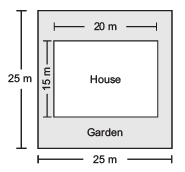Solution: ∵ The given plot is a square with side as 25 m.

∴ Area of the plot = Side * Side

= 25 m * 25 m = 625 m2

∵ The constructed portion is a rectangle having length = 20 m and breadth = 15 m.

∴ Area of the constructed portion = 20 m * 15 m = 300 m2

Now area of the garden = [Total plot area] – [Total constructed area]

= (625 – 300) m2 = 325 m2

∴ Cost of developing the garden = Rs 55 * 325 = Rs 17,875

Question 3. The shape of a garden is rectangular in the middle and semi-circular at the ends as shown in the diagram. Find the area and the perimeter of this garden. [Length of rectangle is 20 – (3.5 + 3.5) metres.]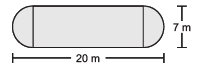Solution: For the semi-circular part:

Diameter of the semi-circle = 7 m

∴ Radius of the semi-circle = 7/2 = 3.5 m

Area of the 2 semi-circles =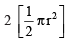= πr2 = 22/7 *(3.5 m)2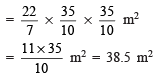Also, perimeter of the 2 semicircles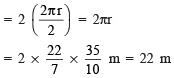For rectangular part:

Length of the rectangle = 20 – (3.5 + 3.5) m = 13 m

Breadth of the rectangle = 7 m

∴ Area = Length * Breadth

= 13 m * 7 m = 91 m2

Perimeter = 2 * [Length + Breadth]

= 2 * [13 m + 0 m]

= 2 * 13 m = 26 m

Now, Area of the garden = (38.5 + 91) m2

= 129.5 m2

Perimeter of the garden = 22 m + 26 m = 48 m

Question 4. A flooring tile has the shape of a parallelogram whose base is 24 cm and the corresponding height is 10 cm. How many such tiles area required to cover a floor of area 1080 m2? (If required you can split the tiles in whatever way want to fill up the corners.)

Solution: Area of a parallelograms = Base * Corresponding height

Area of a tile =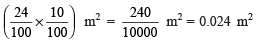∴ Area of the floor = 1080 m2

Now, number of tiles = Total area/Area of one tiles

= 1080/0.024 = 45000 tiles

Question 5. An ant is moving around a few food pieces of different shapes scattered on the floor. For which food-piece would the ant have to take a longer round? Remember, circumference of a circle can be obtained by using the expression c = 2pr, where r is the radius of the circle.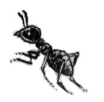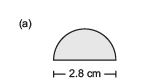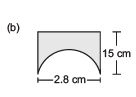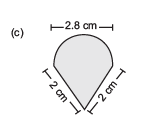Solution: (a) Diameter = 2.8 cm ﬁ Radius = 2.8/2 cm = 1.4 cm

Perimeter of a circle = 2pr ⇒ Perimeter of a semicircle = 2πR/2 = πr

∴  Perimeter of the figure = πr + Diameter

= 22/7 * 1.4 cm + 2.8 cm

= 22/7* 14/10 cm + 2.8 cm

= 4.4 cm + 2.8 cm = 7.2 cm

(b) Perimeter of the semi-circular part = πr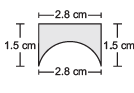= 22/7 * 1.4 cm

= 4.4 cm

Perimeter of the remaining part = 1.5 cm + 2.8 cm + 1.5 cm= 5.8 cm

∴ Perimeter of the figure = 4.4 cm + 5.8 cm = 10.2 cm

(c) Perimeter of the semi-circular part = πd/z  =  22/7 * 2.8/2  = 4.4 cm

∴ Perimeter of the figure = 4.4 cm + 2 cm + 2 cm

= 8.4 cm

Since, 7.2 cm < 8.4 cm < 10.2 cm

∴ Perimeter of figure ‘b’ has the longest round.

Note: In figures ‘b’ and ‘c’, the diameters are not part of the figures.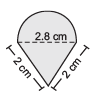Area of Trapezium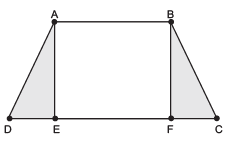The area of a trapezium is the half of the sum of the length of parallel sides and then multiply the perpendicular distances between then.

Here ABCD is a trapezium.

Here AB and CD are parallel sides.

Also AE and BF are perpendicular distance between them.

∴  Area of trapezium of ABCD = 1/2  (AB + CD) * AE

Question 1. Nazma’s sister also has a trapezium shaped plot. Divide it into three parts as shown. Show that the area of trapezium WXYZ =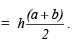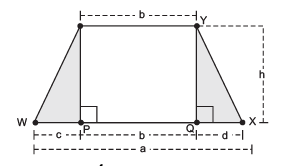Solution:

Area of Δ PWZ =1/2 * Base * Altitude

= 1/2 * c * h = 1/2 ch

Area of the rectangle PQYZ = b * h = bh

Area of Δ QXY = 1/2 * d * h = 1/2 dh

∴ Area of the trapezium XYZW = 1/2 ch + bh +1/2 dh                [∵ c + d + b = a]

= 1/2 (c+d)h + bh       [∵ c + d = a – b]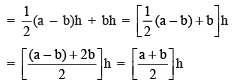Question 2. If h = 10 cm, c = 6 cm, b = 12 cm, d = 4 cm, find the values of each of its parts separately and add to find the area WXYZ. Verify it by putting the values of h, a and b in the expression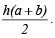Solution: Area of Δ PWZ = 1/2 ch =1/2 * 6 * 10 cm2 = 30 cm2

Area of Δ QXY = 1/2 dh = 1/2 * 4 * 10 cm2 = 20 cm2

Area of rectangle PQYZ = Length * Breadth = b * h

= 12 * 10 cm2 = 120 cm2

\ Area of trapezium WXYZ = Area of Δ PWZ + Area of Δ QXY + Area of rectangle PQYZ

= 30 cm2 + 20 cm2 + 120 cm2 = 170 cm2

Also, area of the trapezium WXYZ =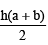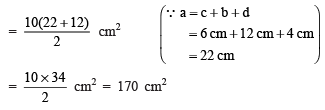Hence, the area of trapezium is verified.

Question: Find the area of the following trapeziums.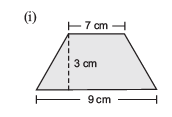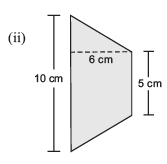Solution:

Note: Area of a trapezium = 1/2* [sum of the parallel sides]*[Perpendicular distance between the parallel sides]

(i) Area of the given trapezium

= 1/2 * (Sum of parallel sides) * (Perpendicular distance between the parallel sides)

= 1/2 * (9 + 7) cm * 3 cm

= 1/2 * 16 cm * 3 cm = 24 cm2

(ii) Area of the given trapezium

= 1/2 * (Sum of the parallel sides) * (Perpendicular distance between the parallel sides)

= 1/2 * (10 + 5) cm * 6 cm

= 1/2 * 15 cm * 6 cm = 45 cm2

Let ABCD be a quadrilateral. Join its vertices A and C such that AC is a diagonal.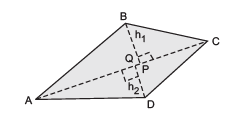Draw BP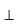AC and DQAC.

Let BP = h1 and DQ = h2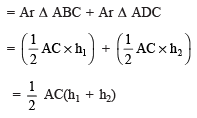[Area of a quadrilateral] = 1/2 * (One of the diagonals) *(Sum of the perpendicular drawn on the diagonal from the opposite vertices)

Example 1. Find the area the quadrilateral LMNO (as shown in the figure).

Solution: Diagonal LN = 8 cm

Perpendicular MX = 4.5 cm

Perpendicular OY = 3.5 cm

∵ Sum of the perpendiculars = MX + OY = 4.5 cm + 3.5 cm = 8 cm

∴  Area of the quadrilateral =1/2 * (A diagonal) * (Sum of the lengths of the perpendiculars on it from opposite vertices)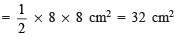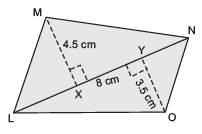Offer running on EduRev: Apply code STAYHOME200 to get INR 200 off on our premium plan EduRev Infinity!

,

,

,

,

,

,

,

,

,

,

,

,

,

,

,

,

,

,

,

,

,

;